#### Value iteration for discounted cost

A backward value iteration solution will be presented that follows naturally from the method given in Section 10.2.1. For notational convenience, let the first stage be designated as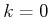so that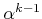may be replaced by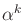. In the probabilistic case, the expected optimal cost-to-go is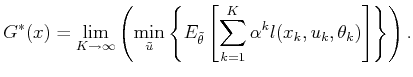(10.69)

The expectation is taken over all nature histories, each of which is an infinite sequence of nature actions. The corresponding expression for the nondeterministic case is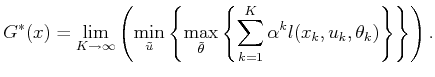(10.70)

Since the probabilistic case is more common, it will be covered here. The nondeterministic version is handled in a similar way (see Exercise 17). As before, backward value iterations will be performed because they are simpler to express. The discount factor causes a minor complication that must be fixed to make the dynamic programming recurrence work properly.

One difficulty is that the stage index now appears in the cost function, in the form of. This means that the shift-invariant property from Section 2.3.1 is no longer preserved. We must therefore be careful about assigning stage indices. This is a problem because for backward value iteration the final stage index has been unknown and unimportant.

Consider a sequence of discounted decision-making problems, by increasing the maximum stage index: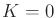,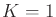,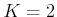,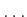. Look at the neighboring cost expressions in Figure 10.10. What is the difference between finding the optimal cost-to-go for the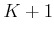-stage problem and the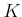-stage problem? In Figure 10.10 the last four terms of the cost for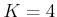can be obtained by multiplying all terms for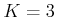by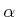and adding a new term,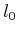. The only difference is that the stage indices need to be shifted by one on each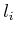that was borrowed from thecase. In general, the optimal costs of a-stage optimization problem can serve as the optimal costs of the-stage problem if they are first multiplied by. The-stage optimization problem can be solved by optimizing over the sum of the first-stage cost plus the optimal cost for the-stage problem, discounted by.

This can be derived using straightforward dynamic programming arguments as follows. Suppose thatis fixed. The cost-to-go can be expressed recursively forfrom 0 toas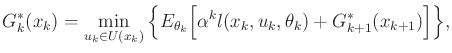(10.71)

in which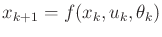. The problem, however, is that the recursion depends onthrough, which makes it appear nonstationary.

The idea of using neighboring cost values as shown in Figure 10.10 can be applied by making a notational change. Let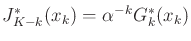. This reverses the direction of the stage indices to avoid specifying the final stage and also scales by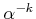to correctly compensate for the index change. Substitution into (10.72) yields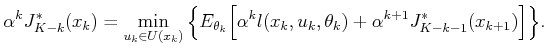(10.72)

Dividing byand then letting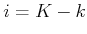yields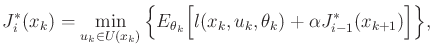(10.73)

in which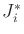represents the expected cost for a finite-horizon discounted problem in which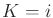. Note that (10.74) expressesin terms of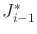, but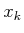is given, and the right-hand side uses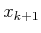. The indices appear to run in opposite directions because this is simply backward value iteration with a notational change that reverses some of the indices. The particular stage indices ofandare not important in (10.74), as long as(for example, the substitutions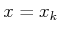,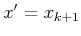,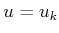, and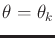can be safely made).

Value iteration proceeds by first letting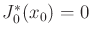for all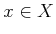. Successive cost-to-go functions are computed by iterating (10.74) over the state space. Under the cycle-avoiding assumptions of Section 10.2.1, the convergence is usually asymptotic due to the infinite horizon. The discounting gradually causes the cost differences to diminish until they are within the desired tolerance. The stationary form of the dynamic programming recurrence, which is obtained in the limit astends to infinity, is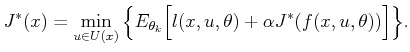(10.74)

If the cost terms do not depend on nature, then the simplified form is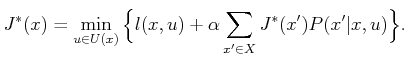(10.75)

As explained in Section 10.2.1, the optimal action,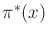, is assigned as the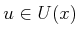that satisfies (10.75) or (10.76) at.

Steven M LaValle 2020-08-14